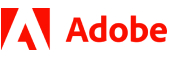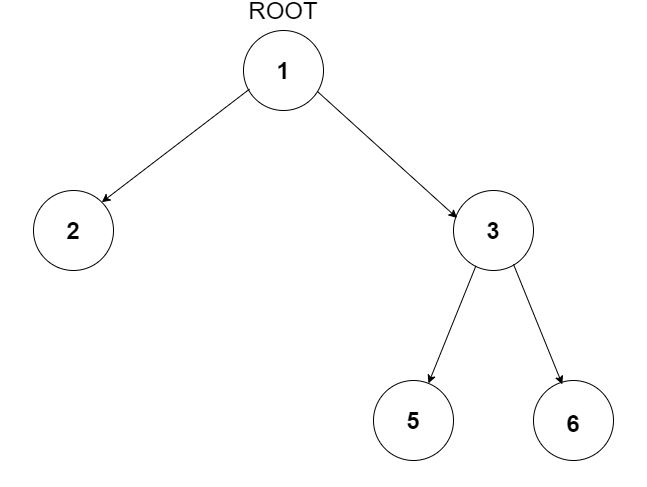New update is available. Click here to update.
Topics

# Triplets in Binary Tree

Easy0/40
Average time to solve is 15m+2 more companies

## Problem statement

You have been given a Binary Tree of integers and an integer 'X'. Find all the triplets in the tree whose sum is strictly greater than 'X'. The nodes in the triplet must hold the relationship of grandparent-parent-child.

For example:
``````For the given binary tree and X = 9
````````````{1, 3, 6} is a valid triplet because 6 is a node whose parent is 3 and grand-parent is 1. Also, the sum of these nodes is 1 + 3 + 6 = 10 which is strictly greater than X = 9.
``````
Detailed explanation ( Input/output format, Notes, Images )
Constraints:
``````1 <= T <= 10 ^ 2
0 <= X <= 10 ^ 9
0 <= N <= 10 ^ 3
-10^9 <= NODE.DATA <= 10^9

Where 'N' is the total number of nodes in the binary tree, and 'NODE.DATA' is the value of a node.

Time Limit: 1 sec.
``````
Sample Input 1:
``````3
9
1 2 3 4 -1 5 6 -1 7 -1 -1 -1 -1 -1 -1
5
1 2 3 -1 -1 -1 -1
2
1 2 -1 3 -1 -1 -1
``````
Sample Output 1:
``````1 3 6
2 4 7
1 2 3
``````
Explanation of Sample Input 1:
``````For the first test case, 6 is a node whose parent is 3 and grand-parent is 1.  is a valid triplet with sum 1 + 3 + 6 = 10 which is greater than 9. Similarly (2,4,7) is also a valid triplet.
````````````For the second test case, there are no valid triplets so don't print anything.

For the third test case, (1, 2, 3) is a valid triplet.
``````
Sample Input 2:
``````1
234
90 84 88 37 87 69 28 -1 -1 -1 -1 -1 -1 -1 -1
``````
Sample Output 2:
``````90 88 69
90 84 87
``````Console